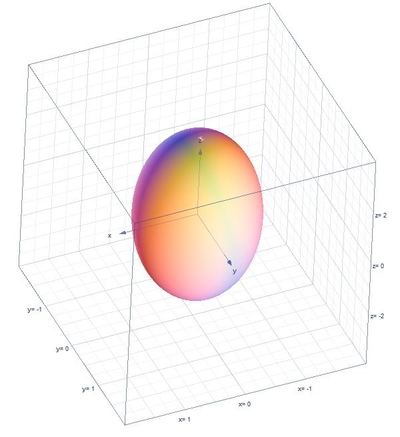# Use a triple integral in cylindrical coordinates to find the volume of the ellipsoid 9x^2 + 9y^2...

## Question:

Use a triple integral in cylindrical coordinates to find the volume of the ellipsoid {eq}\displaystyle 9x^2 + 9y^2 + z^2 = 9. {/eq}

Hint = Integrate over the top half and multiply by 2.

## Volume of the Region:

Solving the volume of the region using the triple integrals in cylindrical coordinates the formula is {eq}V=\int_{\alpha }^{\beta }\int_{h_{1}(\theta )}^{h_{2}(\theta )}\int_{u_{1}(r\cos \theta ,r\sin \theta )}^{u_{2}(r\cos \theta ,r\sin \theta )}r\:f\left ( r\cos \theta ,r\sin \theta ,z \right )dzdrd\theta {/eq} where the conversion formulas are the following {eq}r^{2}=x^{2}+y^{2},\:x=r\cos \theta ,\:y=r\sin \theta {/eq}.

## Answer and Explanation:

Below is the graph,For the upper half of the region our limits would be,

{eq}0\leq \theta \leq 2\pi ,\:0\leq r\leq 1,\:0\leq z\leq \sqrt{9-9r^{2}} {/eq}

The volume of the region is,

{eq}V=2\int_{0}^{2\pi }\int_{0}^{1}\int_{0}^{ \sqrt{9-9r^{2}}}rdzdrd\theta {/eq}

Integrate with respect to {eq}z {/eq}

{eq}V=2\int_{0}^{2\pi }\int_{0}^{1}\left [ z \right ]^{\sqrt{9-9r^{2}}}_{0}rdrd\theta {/eq}

{eq}V=2\int_{0}^{2\pi }\int_{0}^{1}\left ( \sqrt{9-9r^{2}} \right )rdrd\theta {/eq}

Integrate with respect to {eq}r {/eq}

{eq}V=2\int_{0}^{2\pi }\left [ -\left(1-r^2\right)^{\frac{3}{2}} \right ]^{1}_{0}d\theta {/eq}

{eq}V=2\int_{0}^{2\pi }(1)d\theta {/eq}

Integrate with respect to {eq}\theta {/eq}

{eq}V=2\left [ \theta \right ]^{2\pi }_{0} {/eq}

{eq}V=4\pi {/eq}

#### Learn more about this topic:Volumes of Shapes: Definition & Examples

from GMAT Prep: Tutoring Solution

Chapter 11 / Lesson 9
45K